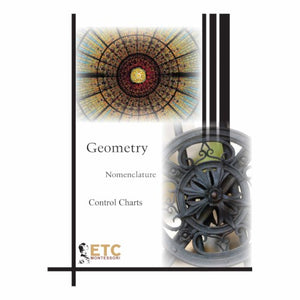# Geometry Nomenclat.:Control Charts Only

Vendor
Nienhuis
Regular price
R 2,835.99
Sale price
R 2,835.99
Regular price
R 2,835.99
Sold out
Unit price
per
Tax included. Shipping calculated at checkout.

Make sure that your upper elementary students have the materials they need to understand essential mathematical concepts. Here is a new resource that allows them to have key geometric nomenclature concepts at their fingertips. Starting with "Point to Solid" and going all the way through to "Parts of Circles", this kit contains 36 control charts for the geometry nomenclature. Printed on cover stock, every picture is in full color and each chart measures 8.5" x 11". Contains the following Charts:
• Point to Solid
• Lines
• Types of Lines
• Parts of a Line & Ray
• Relationship of Line to a Plane
• Relationship Between two Lines
• Axis of a Line Segment
• Angle
• Principle Angles
• Parts of an Angle
• Relationship Between Two Angles
• Combinations of Angles
• Parallel Lines and Two Angle Relationships
• Angle Bisector
• Parts of a Triangle
• Sides of a Right Angled Triangle
• Types of Right Angled Triangles
• Triangles Classified by Sides
• Triangles Classified by Angles
• Closed Curved Regions
• Plane Figures
• Parts of a Circle
• Relationship of a Straight Line to a Circle
• Relative Positions between Circles
• Irregular and Regular Polygons
• Polygons • Parts of a Polygon
• Regular Polygons